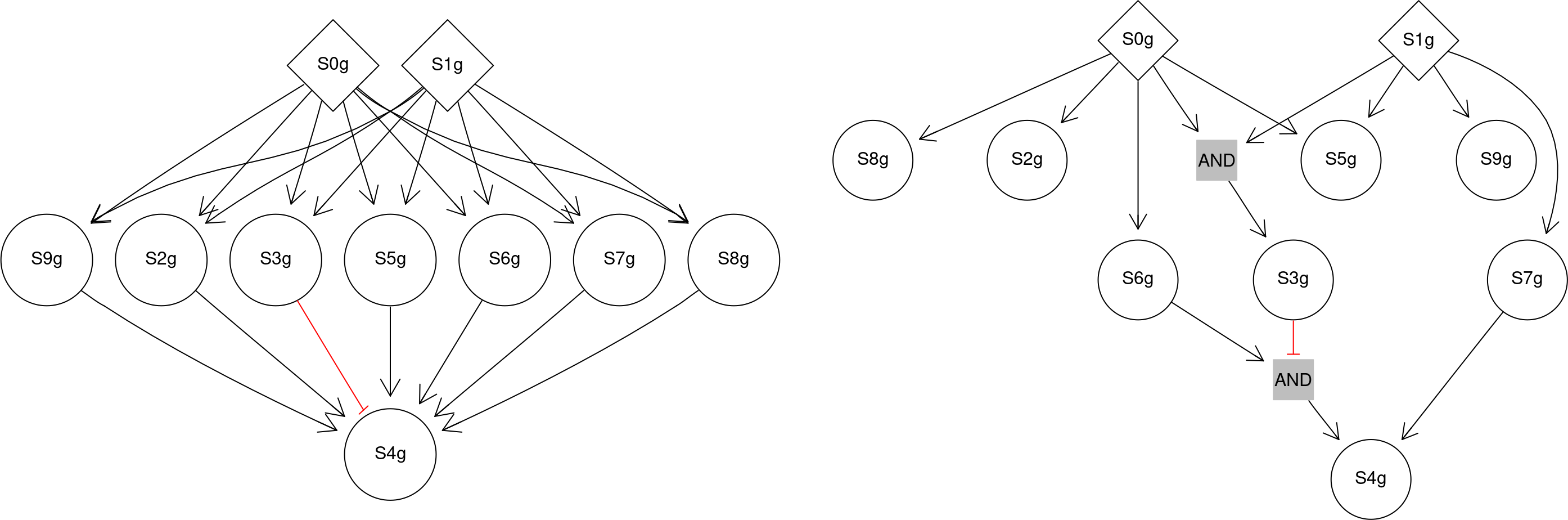# 1 Introduction

Boolean Nested Effects Models (B-NEM) are used to infer signalling pathways. In different experiments (conditions) genes of a pathway (S-genes) are stimulated or inhibited, alone or in combination. In each experiment transcriptional targets (E-genes) of the pathway react differently and are higher or lower expressed depending on the condition. From these differential expressions B-NEM infers Boolean functions presented as hyper-edges of a hyper-graph connecting parents and children in the pathway. For example, if the signal is transduced by two parents A and B to a child C and the signal can be blocked with a knock-down of either one, they are connected by a typical AND-gate. If the signal is still transduced during a single knock-down, but blocked by the double knock-down of A and B, they activate C by an OR-gate. In general the state of child C is defined by a Boolean function $f: \left\{0,1\right\}^n \to \left\{0,1\right\},~C = f(A_1,\dots,A_n)$ with its parents $$A_i, i \in \left\{1,\dots,n\right\}$$.

The B-NEM package is based on and uses many functions of the CellNOptR package of Terfve et al., 2012.

Install the package with the devtools library or via Bioconductor.

if (!requireNamespace("BiocManager", quietly = TRUE)) {
install.packages("BiocManager")
}
BiocManager::install("bnem")

Load the Boolean Nested Effects model package.

library(bnem)

# 2 Toy example for a DAG

We show how to use B-NEM on a toy pathway presented by a directed acyclic (hyper-)graph (DAG). B-NEM demands several objects as input. The two main objects are the differential gene expression (data) and prior knowledge respectively the search space.

The following function creates a DAG, which is then extended to a full Boolean network. From this network we sample a subset of edges to define our ground truth. In the last step, the function generates data given the ground truth.

set.seed(9247)
sim <- simBoolGtn(Sgenes = 10, maxEdges = 10, keepsif = TRUE, negation=0.1,
layer=1)
expression <- sim$expression CNOlist <- sim$CNOlist
model <- sim$model bString <- sim$bString
ERS <- sim$ERS PKN <- sim$PKN
children <- unique(gsub(".*=|!", "", PKN$reacID)) stimuli <- unique(gsub("=.*|!", "", PKN$reacID))
stimuli <- stimuli[which(!(stimuli %in% children))]

The following figure shows the PKN and the ground truth network.

library(mnem)
par(mfrow=c(1,2))
plotDnf(PKN$reacID, stimuli = stimuli) ## A graphNEL graph with directed edges ## Number of Nodes = 10 ## Number of Edges = 21 plotDnf(model$reacID[as.logical(sim$bString)], stimuli = stimuli)Figure 1: PKN and GTN The prior knowledge network without any logics (PKN, left) and the ground truth (GTN, right). Red ‘tee’ arrows depict repression the others activation of the child. The stimulated S-genes are diamond shaped. Blue diamond edges in the PKN depict the existence of activation and repression in one arrow. ## A graphNEL graph with directed edges ## Number of Nodes = 12 ## Number of Edges = 14 We suggest to take a look at the sif file in the working directory. In future analyses it is easier to just provide a suitable sif file for the investigated pathway. In a real application the underlying ground truth network (GTN) is not known. B-NEM uses differential expression between experiments to infer the pathway logics. For example look at the colnames of sim$fc (=foldchanges of E-genes (rows)) and remember that S0g, S1g are our stimulated S-genes and the rest possibly inhibited. Thus in the first column of fc we have the contrast S2g $$-$$ control. In the control no S-genes are perturbed.

We search for the GTN in our restricted network space. Each network is a sub-graph of the full hyper-graph sim$model$reacID. We initialize the search with a starting network and greedily search the neighborhood.

We use two different network scores. “s” is the rank correlation and “cosine” is the cosine similarity (hence no shift invariance).

## we start with the empty graph:
initBstring <- reduceGraph(rep(0, length(model$reacID)), model, CNOlist) ## or a fully connected graph: ## initBstring <- reduceGraph(rep(1, length(model$reacID)), model, CNOlist)

## paralellize for several threads on one machine or multiple machines
## see package "snowfall" for details
parallel <- NULL # NULL for serialization
## or distribute to 30 threads on four different machines:
## parallel <- list(c(4,16,8,2), c("machine1", "machine2", "machine3",
## "machine4"))

## greedy search:
greedy <- bnem(search = "greedy",
fc=fc,
expression=expression, # not used, if fc is defined
CNOlist=CNOlist,
model=model,
parallel=parallel,
initBstring=initBstring,
draw = FALSE, # TRUE: draw network at each step
verbose = FALSE, # TRUE: print changed (hyper-)edges and
## score improvement
maxSteps = Inf,
method = "s"
)

greedy2 <- bnem(search = "greedy",
fc=fc,
expression=expression,
CNOlist=CNOlist,
model=model,
parallel=parallel,
initBstring=initBstring,
draw = FALSE,
verbose = FALSE,
maxSteps = Inf,
method = "cosine"
)

We compare the binary strings defining the ground truth and the inferred networks. We first look at the respective scores (the smaller the better) and then compute sensitivity and specificity of the edges for both results.

greedy$scores # rank correlation = normalized ## [] ##  -31.96792 -34.09256 -35.49737 -36.50558 -37.35063 -38.17197 -38.94132 ##  -39.47262 -39.97767 -40.25966 -40.52911 -40.59341 -40.66502 greedy2$scores # scaled log foldchanges
## []
##   -32.52366 -34.89870 -36.05350 -37.09127 -37.94433 -38.79064 -39.53877
##   -40.01382 -40.40855 -40.78858 -40.96302 -41.06156 -41.08302
accuracy <- function(gtn,inferred) {
sens <- sum(gtn == 1 & inferred == 1)/
(sum(gtn == 1 & inferred == 1) + sum(gtn == 1 & inferred == 0))
spec <- sum(gtn == 0 & inferred == 0)/
(sum(gtn == 0 & inferred == 0) + sum(gtn == 0 & inferred == 1))
return(c(sens,spec))
}

accuracy(bString,greedy$bString) ##  0.9000000 0.9487179 accuracy(bString,greedy2$bString)
##  0.9000000 0.9230769
resString <- greedy2$bString We take a look at the efficiency of the search algorithm with sensitivity and specificity of the hyper-edges for the optimized network and the accuracy of its ERS (similar to the truth table). Since several networks produce the same ERS, the learned hyper-graph can differ from the GTN and still be $$100\%$$ accurate. par(mfrow=c(1,2)) ## GTN: plotDnf(model$reacID[as.logical(bString)], main = "GTN", stimuli = stimuli)
## A graphNEL graph with directed edges
## Number of Nodes = 12
## Number of Edges = 14
## greedy optimum:
plotDnf(model\$reacID[as.logical(resString)], main = "greedy optimum",
stimuli = stimuli)